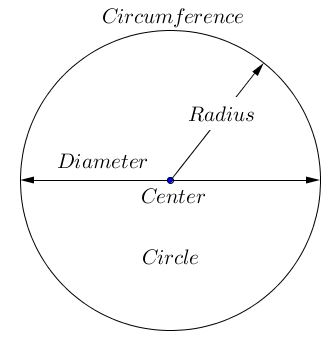# C Program Find Circumference Of A Circle | 3 Ways

C Program to find the Circumference of a Circle – In this specific article, we will brief in on the methods of finding the circumference of a circle in C Programming. With the help of this piece, we will explain all the types of ways the circumference of a circle can be calculated. The various ways by which the circumference is calculated in the C program are as follows:

• Using Standard Method
• Using Function
• Using Pointers
• Using Macros

Circles, as we all know, is the locus of a collection of points which form the boundary and are equidistant from a single point in the middle. The boundary of a circle is known as the Circumference of a circle.

All the points that form the boundary or the circumference of the circle are equidistant from a single point inside the circle.

The single point that is present inside the circle is also known as the Center of the circle. The distance from the Center of a circle to any point on the circumference is equal. It is regarded as the Radius of that very circle.

If we expand the Radius on the opposite direction and create a line that connects both sides of the circumference while going through the Center, it is known as the Diameter of the circle.

Let us see what all of the above look like in a pictorial form:As we can see, the four most important aspects of a circle are given in the image uploaded above. Those are as follows:

• Center
• Circumference
• Diameter

The radius of a circle is denoted with “r”. The diameter of a circle is denoted with “d”. It is known that the diameter of a circle is double that of the radius.

Mathematically,

d = 2r

Also, the ratio between the circumference and the diameter of a circle is equal to the value of Pi (π). So, we can deduce the formula to calculate the circumference of a circle from this very line.

C/d = π or 22/7

=> C = dπ

=> C = 2πr (since d = 2r)

As we can see, this is the correct formula to find the value of the circumference of any circle. Henceforth, this formula will be used in order to calculate the circumference of a circle in the C programs. The various types of C programs are as follows:

## Using Standard Method

1. The formula for the circumference of a circle is c=(2*22*r)/7.
2. The radius value will store into the variable “r”.
3. By substituting the “r ” value into the formula we will get circumference value, that value will store into the variable “c”.
Output:

### Using Function

1. Here we using the function float area(int r) to calculate the area of the circumference circle.
2. We will call the function using area(r), here we are passing the value of “r”.
3. The called function “area(int r)” will calculate the area of the circumference and returns the value, the return value will be assigned to the variable “a”.
Output:

#### Using Pointers

1. We are calling the function by passing addresses as arguments in area(&r,&c).
2. The function void area(float *r, float *a) will calculate the area of the circumference which is having pointer arguments.
3. The calculated value will be stored into the variable a.
Output:
##### Using Macros
1. line 2 represents area(r) is a symbolic name for the expression (2*22*r)/7
2. area(r)replaced with that expression given at #define.
Output:
x

## C Program To Check If Alphabet, Digit or Special Character | C Programs

C program to check for an alphabet, digit or special character – In this article, ...